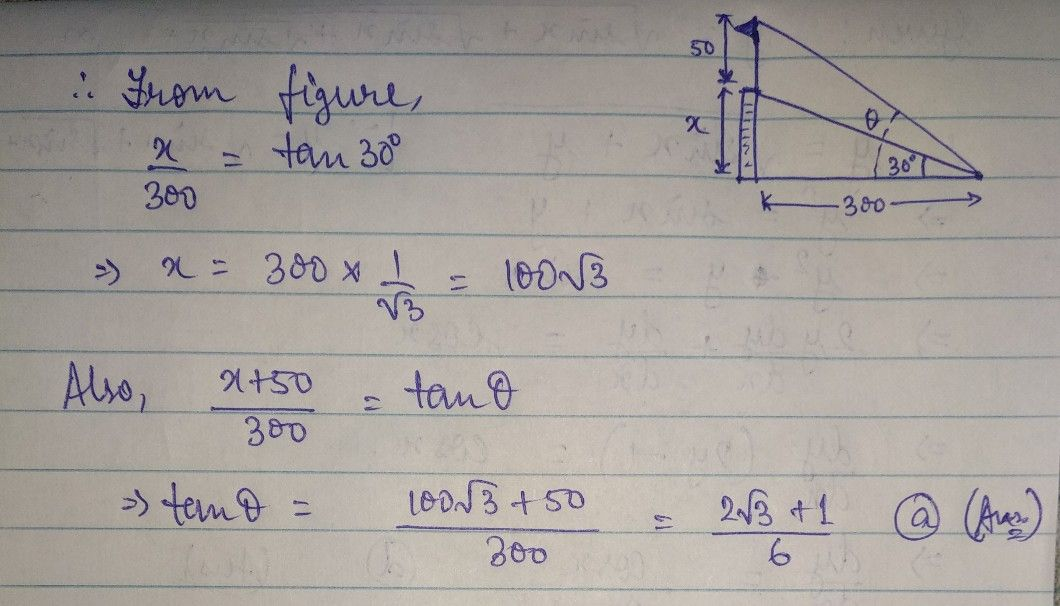Symbol
Problem$A$ A flagpole stands on a building and $a∩$ observer on a level ground is $300$ feet $xOm$ the base of the building. The angle $0f$ $eleVati0n$ of the bottom of the flagpole is $30^{°}$ and the height of the flagpole is $00$ feet. If $θ$ 0 is the angle of elevation of the top of the flagpole, then tan $θ$ is equal to $\left(a\right)$ $\dfrac {2\sqrt{3} +1} {6}$ (b) $\dfrac {\sqrt{3} } {2}$ $\left(c\right)$ $1$ Ka $\left(d\right)$ $\dfrac {6\sqrt{3} +1} {6}$